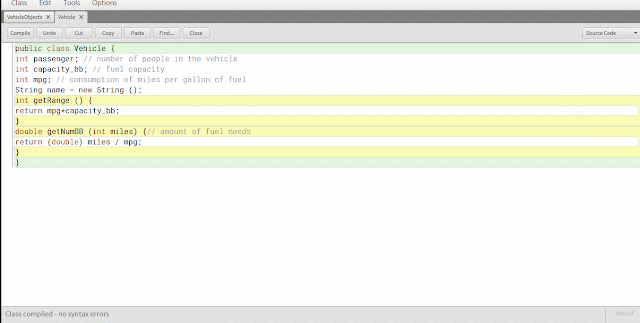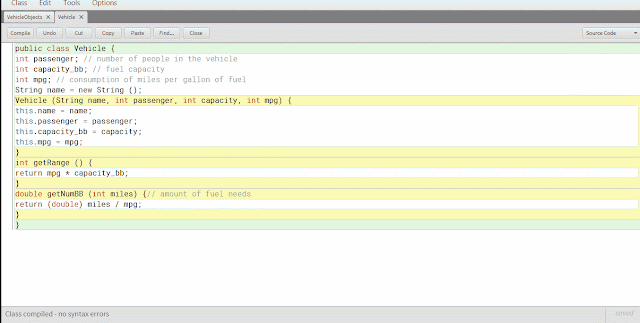# Object, Class, Message and Method JAVA

### Object, Class, Message and Method

In object-oriented programming, a class is a template for structure definition and object creation. So the object of an instance of a class, that class is a collection of plans that specify how to build an object.

#### Object and Class

Information-related information and describes an object called object attributes (object attribute). This attribute allows an object has a value attribute that stands alone. Besides object attributes also have an action called by the method. So the method is a set of operations on an object in the form of code instructions.
General syntax format-making class is as follows:

class classname {
// declare instance variables var1 type;
var2 type;
// ... type varN;
// declare methods
type method1 (parameters) {
// body of method
}
type method2 (parameters) {
// body of method
}
// ...
type methodN (parameters) {
// body of method
}
}

#### Defining Class

Consider the example of a class definition of vehicles consisting of three instance variables and the making of objects of that class, such as vehicle class pembuaan below:
 `1` `public ``class` `Vehicle {`
 `2` `int` `passenger; ``/``/` `number of people ``in` `the vehicle`
 `3` `int` `capacity_bb; ``/``/` `fuel capacity`
 `4` `int` `mpg; ``/``/` `consumption of miles per gallon of fuel`
 `5` `}`

#### Creating Objects

Objects created by the pre-defined class that is a class above the previous vehicle.
so coding for the object as follows:

 `01` `public ``class` `VehicleObjects {`
 `02` `public static void main (String [] args) {`
 `03` `Vehicle minivan ``=` `new Vehicle ();`
 `04` `Vehicle sportscar ``=` `new Vehicle ();`
 `05` `int` `range1, range2;`
 `06` `minivan.passenger ``=` `7``;`
 `07` `minivan.capacity_bb ``=` `16``;`
 `08` `minivan.mpg ``=` `21``;`
 `09` `sportscar.passenger ``=` `2``;`
 `10` `sportscar.capacity_bb ``=` `14``;`
 `11` `sportscar.mpg ``=` `12``;`
 `12` `range1 ``=` `minivan. capacity_bb ``*` `minivan.mpg;`
 `13` `range2 ``=` `sportscar. capacity_bb ``*` `sportscar.mpg;`
 `14` `System.out.println (``"Minivan can carry "`
 `15` `+` `minivan. passenger ``+` `" person with range "` `+` `range1);`
 `16` `System.out.println (``"Sportscar can bring "`
 `17` `+` `sportscar. passenger ``+` `" person with range "` `+` `range2);`
 `18` `}`
 `19` `}`coding results

Class that has been made over there just an instance variable, now add some operation (method) into the class.
vehicle class with the method:

 `01` `public ``class` `Vehicle {`
 `02` `int` `passenger; ``/``/` `number of people ``in` `the vehicle`
 `03` `int` `capacity_bb; ``/``/` `fuel capacity`
 `04` `int` `mpg; ``/``/` `consumption of miles per gallon of fuel`
 `05` `String name ``=` `new String ();`
 `06` `int` `getRange () {`
 `07` `return` `mpg``*``capacity_bb;`
 `08` `}`
 `09` `double getNumBB (``int` `miles) {``/``/` `amount of fuel needs`
 `10` `return` `(double) miles ``/` `mpg;`
 `11` `}`
 `12` `}`

objekvehicle class with the method:

 `01` `public ``class` `VehicleObjects {`
 `02` `public static void main (String [] args) {`
 `03` `Vehicle minivan ``=` `new Vehicle ();`
 `04` `Vehicle sportscar ``=` `new Vehicle ();`
 `05` `double gallon;`
 `06` `int` `distance ``=` `259``;`
 `07` `minivan.name ``=` `"Minivan"``;`
 `08` `minivan.passenger ``=` `7``;`
 `09` `minivan.capacity_bb ``=` `16``;`
 `10` `minivan.mpg ``=` `21``;`
 `11` `sportscar.name ``=` `"Sportscar"``;`
 `12` `sportscar.passenger ``=` `2``;`
 `13` `sportscar.capacity_bb ``=` `14``;`
 `14` `sportscar.mpg ``=` `12``;`
 `15` `gallon ``=` `minivan.getNumBB (distance);`
 `16` `System.out.println (minivan.name ``+` `" requires "` `+` `gallon ``+`
 `17` `" gallons to travel distance "` `+` `distance ``+` `" miles."``);`
 `18` `gallon ``=` `sportscar.getNumBB (distance);`
 `19` `System.out.println (sportscar.name ``+` `" requires "` `+` `gallon ``+`
 `20` `" gallons to travel distance "` `+` `distance ``+` `" miles."``);`
 `21` `}`
 `22` `}`coding results

#### constructor

A constructor is an object initialization when it is created.  Constructor name equal to the name of its class.

vehicle class with a constructor:

 `01` `public ``class` `Vehicle {`
 `02` `int` `passenger; ``/``/` `number of people ``in` `the vehicle`
 `03` `int` `capacity_bb; ``/``/` `fuel capacity`
 `04` `int` `mpg; ``/``/` `consumption of miles per gallon of fuel`
 `05` `String name ``=` `new String ();`
 `06` `Vehicle (String name, ``int` `passenger, ``int` `capacity, ``int` `mpg) {`
 `07` `this.name ``=` `name;`
 `08` `this.passenger ``=` `passenger;`
 `09` `this.capacity_bb ``=` `capacity;`
 `10` `this.mpg ``=` `mpg;`
 `11` `}`
 `12` `int` `getRange () {`
 `13` `return` `mpg ``*` `capacity_bb;`
 `14` `}`
 `15` `double getNumBB (``int` `miles) {``/``/` `amount of fuel needs`
 `16` `return` `(double) miles ``/` `mpg;`
 `17` `}`
 `18` `}`

objekvehicle class with a constructor:
 `01` `public ``class` `VehicleObjects {`
 `02` `public static void main (String [] args) {`
 `03` `Vehicle minivan ``=` `new Vehicle (``"Minivan"``, ``7``,``16``,``21``);`
 `04` `Vehicle sportscar ``=` `new Vehicle (``"Sportscar"``, ``2``,``14``,``12``);`
 `05` `double gallon;`
 `06` `int` `distance ``=` `259``;`
 `07` `gallon ``=` `minivan.getNumBB (distance);`
 `08` `System.out.println (minivan.name ``+` `" requires "` `+` `gallon ``+`
 `09` `" gallons to travel distance "` `+` `distance ``+` `" miles."``);`
 `10` `gallon ``=` `sportscar.getNumBB (distance);`
 `11` `System.out.println (sportscar.name ``+` `" requires "` `+` `gallon ``+`
 `12` `" gallons to travel distance "` `+` `distance ``+` `" miles."``);`
 `13` `}`
 `14` `}`coding results